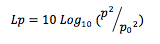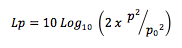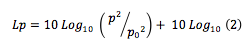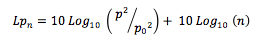# Adding Decibels: Why 1 & 1 = 4

The sound pressure level is given by:If the sound energy is doubled this becomes:Which can be rewritten as:As 10 Log10 (2) is approximately equal to 3 this means that if the sound energy is doubled the sound level increases by 3dB. This holds true regardless of the starting point, so just as 1 dB & 1 dB = 4 dB, so 88 dB & 88 dB = 91 dB.

This can be written more generally as:Where:

n = number of identical sources i.e. proportionate increase in sound energy

So a tripling of sound energy equates to a 5 dB increase (from 10 Log10 (3) = 5) etc.